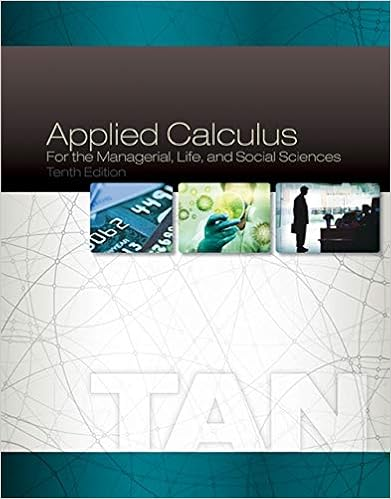# Quiz 3 Key - QUIZ 3 Solution Key Math 141/6380 Due 11:59PM...

• Test Prep
• 3
• 94% (17) 16 out of 17 people found this document helpful

This preview shows page 1 - 2 out of 3 pages.

##### We have textbook solutions for you!
The document you are viewing contains questions related to this textbook.The document you are viewing contains questions related to this textbook.
Chapter 3 / Exercise 5
Applied Calculus for the Managerial, Life, and Social Sciences
TanExpert Verified
QUIZ # 3 Solution Key Math 141/6380, Due 11:59PM, Sunday, June 18, 2017 1. Show that the function ?(𝑥) = ?𝑥3+2𝑥+1is one-to-one. Graphing does not count as proof. (Hint: Use the derivative of the function.)
=
2. Find a formula for the inverse function )(1xfof (𝑥) = 3 − ln(𝑥). Verify that ?−1(?(?7)) = ?7
3. Fill in the values of ?−1(𝑥)and (?−1)(𝑥)for 𝑥 = −1, 1, 2. Provide detail to support your answers.
?
?
?
##### We have textbook solutions for you!
The document you are viewing contains questions related to this textbook.The document you are viewing contains questions related to this textbook.
Chapter 3 / Exercise 5
Applied Calculus for the Managerial, Life, and Social Sciences
TanExpert Verified
•••